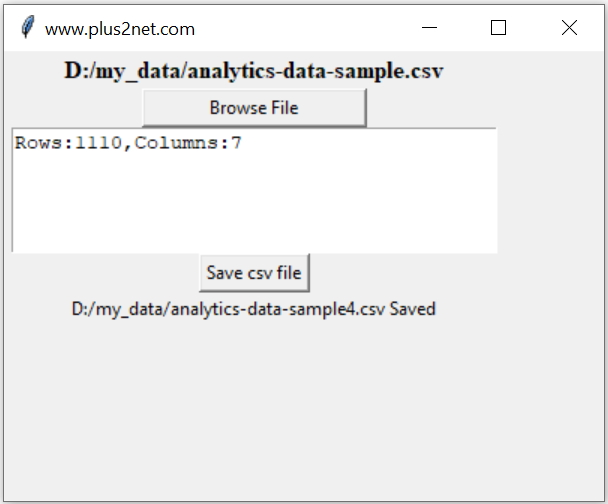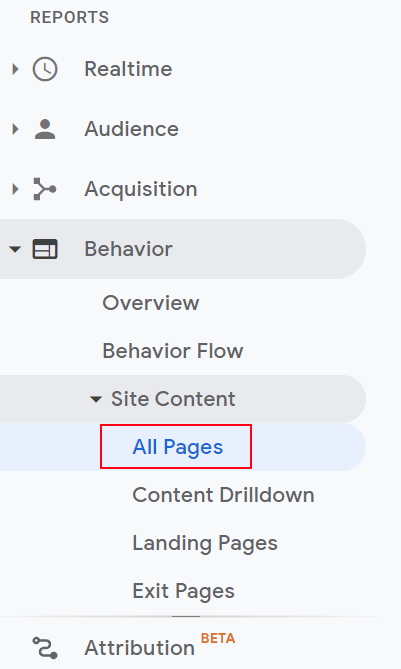# DataFrame from Analytics CSV file using Tkinter GUI

Tkinter Projects in Tkinter PandasDownload data from your google analytics over a period as CSV file ( Comma Separated value ). This script has four parts.

1. Part 1 : Download data from google Analytics and save as CSV file. Or you can use sample file available at end of this page.
2. Part 2 : read the data and create on Pandas DataFrame
3. Part 3 : Clean the Data to match the requirement
4. Part 4 : Save the dataframe as CSV file.

Bahavior > Site Content > All PagesCreate a date range using From date and To date, then select Export and use CSV ( Comma Separated Value ) data as file type.
At the bottom change the show rows option to higher number ( by default it is 10 ) based on number of pages you want to download.## Part II : Read the CSV file and create DataFrame

Use the file browser to connect to any csv ( Comma Separated value ) file on click of a button. Use the read_csv() method to create a Pandas Dataframe by using the selected csv file.
While creating the DataFrame by using read_csv() we have to understand the file structure of the downloaded CSV file. Here the file you download from Google Analytics will have some blank lines at the top and at the end of the page. So we need to skip 6 rows by using the option skiprows.
``df=pd.read_csv(file,skiprows=6)``

After creation of Dataframe , display the number of rows and columns in the dataframe by using DataFrame attribute shape.

## Remove rows with NaN

``df.dropna(subset=['Page'],inplace=True)``

## Delete rows with not matching data length

Remove rows with blank data or not real data based on length. Here any data in Page column having length less than 12 and more than 3 are removed. The second part is added to take care of home page which has 0 length. In all other pages length has to be more than 12.

This condition may change in your cases.
``````my_delete=df[(df['Page'].str.len() <12) & (df['Page'].str.len() >3)].index
df.drop(my_delete,inplace=True,axis=0)``````

## Remove pages with Query string

We don't want multiple rows of same page with different query string. Here to indentify such page we checked for presence of ? and based on this matching we removed the row.
``df=df[~df.Page.str.contains('\?')]``

## Delete column

You can delete any column based on your requirment. Here one column Page Value with data is removed.
``df.drop(labels='Page Value',axis=1,inplace=True)``

## Change the column names

To match with the requirment of any database, we can change the column names by removing space and by adding underscore ( _ ). Maintain the sequence.
``df.columns = ['Page','p_view','u_view','avg','entrance','b_rate','b_exit']``
Create a string using the number of rows and columns of the dataframe. Add the string to Text widget.
``````str1="Rows:" + str(df.shape)+ ",Columns:"+str(df.shape)
t1.insert(tk.END, str1) ``````

## Add button to save the dataframe

On click of a button we will show the file save as dialog box and save the dataframe as csv file.
``````b1=tk.Button(my_w,text='Save csv file',command=lambda:save_file())
b1.grid(row=4,column=1)
def save_file():
filetypes=[("csv file", ".csv")],
defaultextension=".csv")
if file:
df.to_csv(file,index=False) # store as CSV file
l3=tk.Label(my_w,text=file + ' Saved')
l3.grid(row=5,column=1)``````

Subscribe to our YouTube Channel here

## Subscribe

* indicates required
Subscribe to plus2netplus2net.com

Post your comments , suggestion , error , requirements etc here